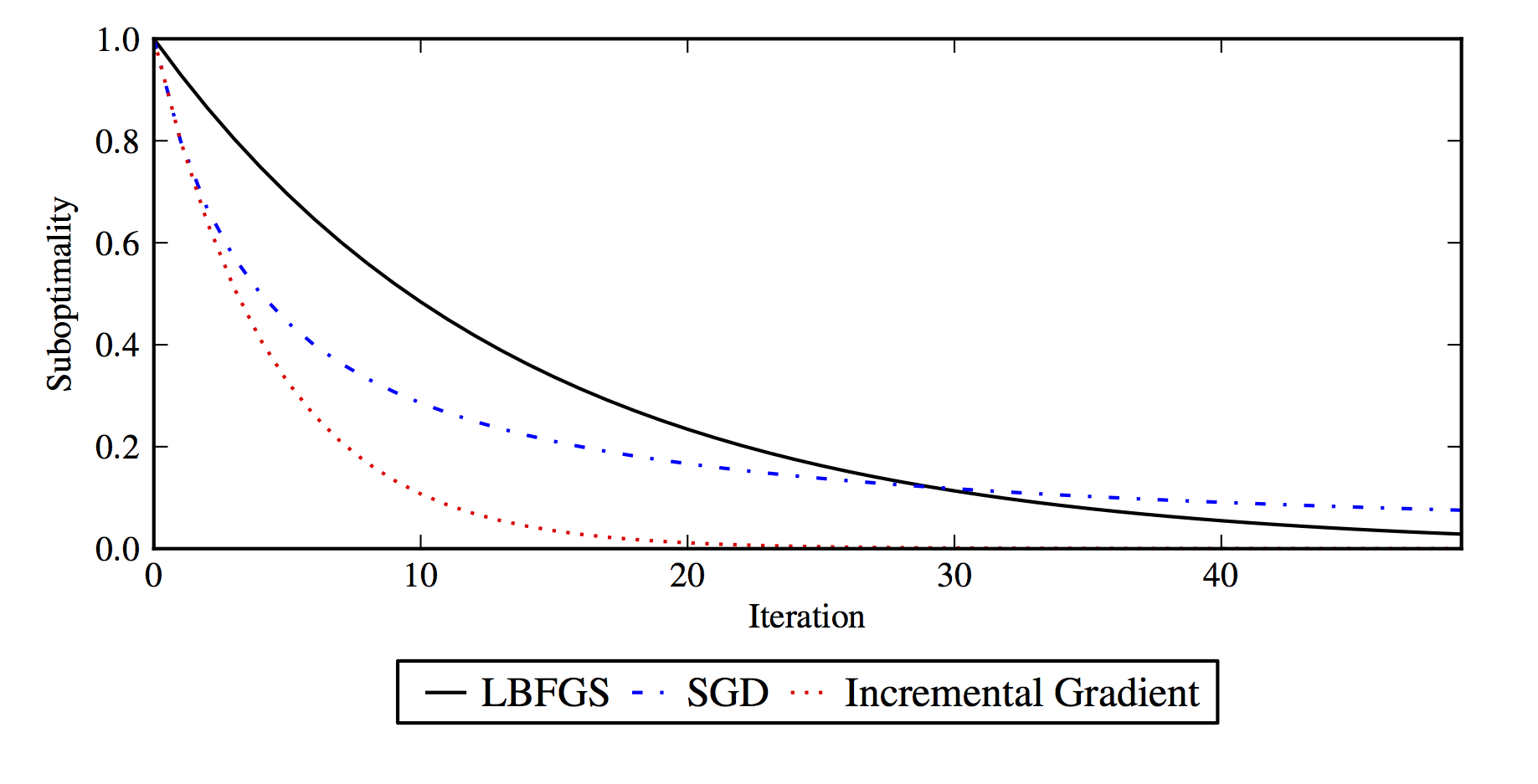# Finally an alternative to SGD: Stochastic variance reduction methods

This post describes the newly developed class of variance reduction techniques in stochastic optimisation, which are fast and simple alternatives to the traditional stochastic gradient descent (SGD) method. I give a light introduction here, focused on actually applying the methods in practice.

### But first: Ye Old SGD

Quite possibly the most important algorithm in machine learning, SGD is simply a method for optimizing objective functions that consist of a sum or average of terms:

$f\left(x\right)=\frac{1}{n}\sum _{i=1}^{n}{f}_{i}\left(x\right).$

The SGD method maintains an estimate ${x}_{k}$ of the solution at step $k$, and at each step:

1. Sample: Pick an index $j$ uniformly at random (a datapoint index).
2. Step: Update $x$:
${x}_{k+1}={x}_{k}-\gamma {f}_{j}^{\prime }\left({x}_{k}\right).$

At it’s most basic, this update is just an approximation of the classical gradient descent technique, where we take the non-random step ${x}_{k+1}={x}_{k}-\gamma {f}^{\prime }\left({x}_{k}\right).$ The step size parameter $\gamma$ here is just a constant, which depending on the problem may need to be adjusted down at each step. Variance reduction methods build upon SGD by modifying the core update in various ways.

### SAGA

The SAGA method is a simple modiﬁcation of SGD, where we remember the last gradient evaluated for each datapoint, and use this to correct the SGD step to reduce its variance. These past gradients are stored in a table. We denote the previous gradient for datapoint j as ${f}_{j}^{\prime }\left({\varphi }_{j}^{k}\right)$. The core step is as follows:

1. Sample: Pick an index $j$ uniformly at random.
2. Step: Update $x$ using ${f}_{j}^{\prime }\left({x}^{k}\right)$, ${f}_{j}^{\prime }\left({\varphi }_{j}^{k}\right)$ and the table average:
 ${x}^{k+1}={x}^{k}-\gamma \left[{f}_{j}^{\prime }\left({x}^{k}\right)-{f}_{j}^{\prime }\left({\varphi }_{j}^{k}\right)+\frac{1}{n}\sum _{i=1}^{n}{f}_{i}^{\prime }\left({\varphi }_{i}^{k}\right)\right],$ (1)

3. Update the table: Denote ${\varphi }_{j}^{k+1}={x}^{k}$, and store ${f}_{j}^{\prime }\left({\varphi }_{j}^{k+1}\right)$ in the table. All other entries in the table remain unchanged. The quantity ${\varphi }_{j}^{k+1}$ is not explicitly stored.

Source code for SAGA and the accelerated variant point-saga is available on github. Some points about the algorithm:

• Storing the past gradients is typically fast and low cost. For logistic regression or least squares, it reduces to storing a single real value per datapoint instead of a full gradient. Similar reductions apply to neural networks. When the storage cost is high, the SVRG method described below can be used instead.
• The table average $\frac{1}{n}{\sum }_{i=1}^{n}{f}_{i}^{\prime }\left({\varphi }_{i}^{k}\right)$ is of course cached rather then recalculated at each step.
• Notice that there is only really two extra terms on top of the usual SGD step. The two terms also trivially cancel when you take the expectation with respect to $j$. So the expected step direction is a gradient step, just like with SGD.
• The step above assumes you already have a table of gradients. If you are just starting the algorithm, do the ﬁrst pass over the data in-order (instead of random), and use the table average over only the data seen so far in the step. Alternatively, just do regular SGD steps during the ﬁrst pass.
• The requirement for randomly sampling datapoints instead of doing in-order passes turns out to be absolutely crucial for it to work.
• Implementing the step eﬃciently when the gradients are sparse is a little subtle, but possible. See the SAGA paper for details and example code.
• SAGA supports non-diﬀerentiable regularisers through the use of proximal operators. See the paper for details.

### Why variance reduction methods?

VR methods like SAGA have entirely diﬀerent convergence properties than SGD. On strongly convex problems they are able to converge linearly ($O\left(log\left(1∕k\right)$), instead of the much slower $O\left(1∕k\right)$ convergence possible with the SGD method (which requires careful iterate averaging). Linear convergence on a stochastic method is quite remarkable, up until recently no such methods were known. It’s also fast linear convergence, compared to the theoretical rate for regular gradient descent, it makes 1/3 the progress each step, but each step is only a single datapoint evaluation rather than a full batch gradient. For large datasets that can be millions of times faster. A schematic illustration of the practical speedup is below, labeled as "incremental gradient":### Other variance reduction techniques

SAGA is perhaps the most straightforward of the variance reduction techniques. The other VR methods have advantages in certain situtations, there is no clear best method in all cases. We list the main methods here, each has several variants as well:

SAG
is the earliest of these VR methods developed. It doesn’t actually take the gradient direction in expectation, rather it introduces a small bias with the potential for a lower variance in the gradient estimate. Unfortunately SAG is hard to extend from the point of view of the theory.
SVRG
is the direct predecessor of SAGA that makes a diﬀerent trade-oﬀ. It avoids storing gradients, but at the expense of computing two gradients per step instead of one. It also has a more complex double loop formulation, where the outer loop requires a recalculation of the full-data gradient. In most cases the extra computation makes it slower than SAGA, but it does have some applications.
Finito/Miso
take a step derived indirectly from the dual formulation of the problem, while avoiding any dual computation. They don’t work eﬃcently on sparse problems, but are perhaps the fastest methods on dense problems.
SDCA
is a purely dual method. It can be applied to some non-diﬀerentiable problems unlike the other methods here, but it is complex to apply eﬃcently to other problems such as logistic regression. It also has the nice properly of avoiding tunable parameters on problems with L2 regularisation. For practical application it crucially requires a non-degenerate amount of strong convexity though.